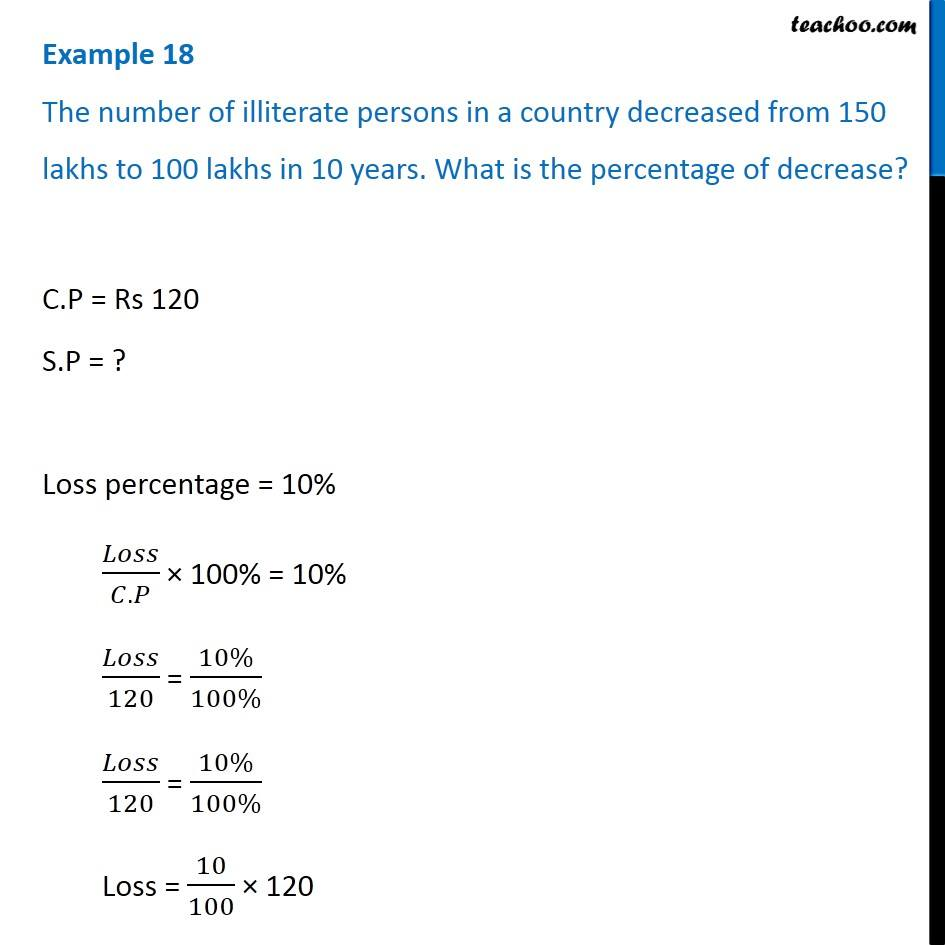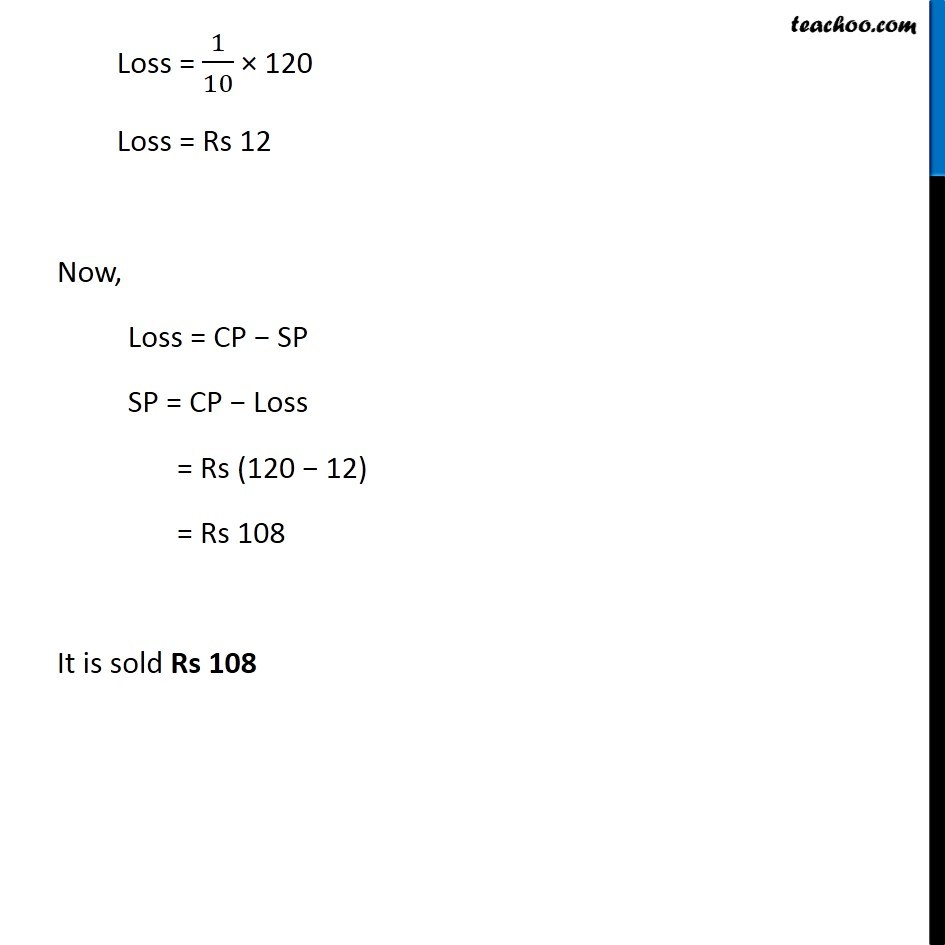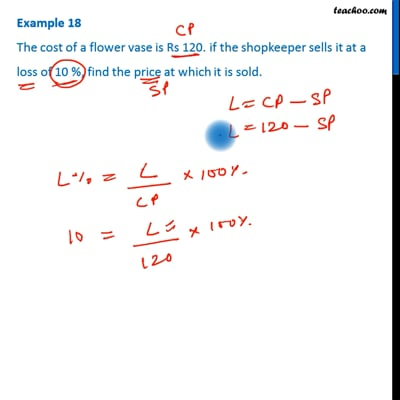Examples

Chapter 8 Class 7 Comparing Quantities
Serial order wiseThis video is only available for Teachoo black users

Get live Maths 1-on-1 Classs - Class 6 to 12

### Transcript

Example 18 The number of illiterate persons in a country decreased from 150 lakhs to 100 lakhs in 10 years. What is the percentage of decrease? C.P = Rs 120 S.P = ? Loss percentage = 10% 𝐿𝑜𝑠𝑠/(𝐶.𝑃) × 100% = 10% 𝐿𝑜𝑠𝑠/120 = (10%)/(100%) 𝐿𝑜𝑠𝑠/120 = (10%)/(100%) Loss = 10/100 × 120 Loss = 1/10 × 120 Loss = Rs 12 Now, Loss = CP − SP SP = CP − Loss = Rs (120 − 12) = Rs 108 It is sold Rs 108